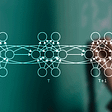# GANs, cGANs, AE, AAE, AVE, CAAE, CAVE

The difference between each other.

## Unconditional GAN

The generator (G) and discriminator (D) are both feedforward neural networks which play a min-max game between one another.

The generator takes as input a vector of random numbers (z), and transforms it into the form of the data we are interested in imitating (G(z)). The discriminator takes as input a set of data, either real (x) or generated (G(z)), and produces a probability of that data being real (P(x)). We would have the objective function min_G max_D L_GAN(G,D)

Optimize discriminator:

The discriminator is optimized in order to increase the likelihood of giving a high probability to the real data and a low probability to the generated data. max(log(p(x_i)+log(1-p(x’_i))). Because p(x_i) in (0, 1] so the log(p(x_i)) is negative, in the code, we define loss_d =reduced_mean(-log(p(x_i)-log(1-p(x’_i))), so we just need the optimizer to find the lowest possible loss.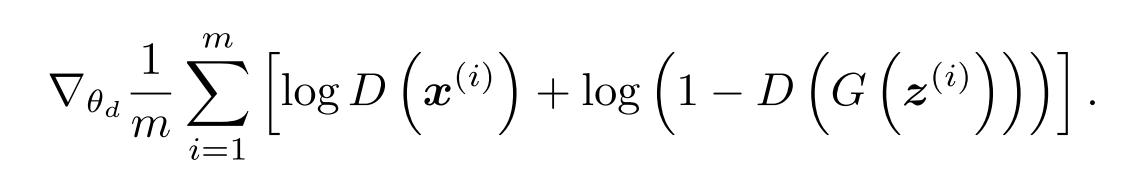The gradient ascent expression for the discriminator. The first term corresponds to optimizing the probability that the real data (x) is rated highly. The second term corresponds to optimizing the probability that the generated data G(z) is rated poorly. Notice we apply the gradient to the discriminator, not the generator.

Optimize generator:

The generator is then optimized in order to increase the probability of the generated data being rated highly. min(log(1-P(x’_i))). In code, this is equivalent to define loss_g =reduce_mean(-log(p(x’_i)).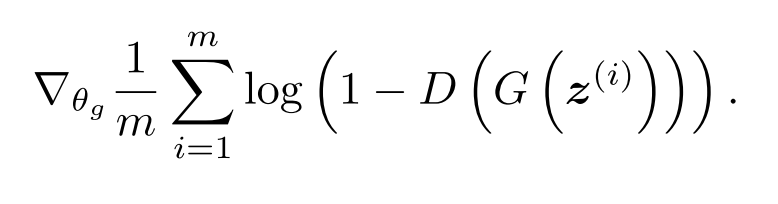The gradient descent expression for the generator. The term corresponds to optimizing the probability that the generated data G(z) is rated highly. Notice we apply this gradient to the generator network, not the discriminator.

By alternating gradient optimization between the two networks using these expressions on new batches of real and generated data each time, the GAN will slowly converge to producing data that is as realistic as the network is capable of modeling. If you are interested, you can read the original paper introducing GANs here for more information.

See it through Code

`with tf.variable_scope('G'):    z = tf.placeholder(tf.float32, shape=(None, 1))    G = generator(z, hidden_size)with tf.variable_scope('D') as scope:    x = tf.placeholder(tf.float32, shape=(None, 1))    D1 = discriminator(x, hidden_size)    scope.reuse_variables()    D2 = discriminator(G, hidden_size)loss_d = tf.reduce_mean(-tf.log(D1) - tf.log(1 - D2))loss_g = tf.reduce_mean(-tf.log(D2))`

## Conditional GAN

Generative adversarial nets can be extended to a conditional model if both the generator and discriminator are conditioned on some extra information y. y could be any kind of auxiliary information, such as class labels or data from other modalities. And in practice, y is encoded as one-hot vector of size n if it has n class labels. We can perform the conditioning by feeding y into the both the discriminator and generator as additional input layer.

Example one: MNIST the noise is uniform distribution.

Example two: Generate user-tags from images

Photo sites such as Flickr are a rich source of labeled data in the form of images and their associated user-generated metadata (UGM) — in particular user-tags.

User-generated metadata differ from more ‘canonical’ image labelling schems in that they are typically more descriptive, and are semantically much closer to how humans describe images with natural language rather than just identifying the objects present in an image. Another aspect of UGM is that synoymy is prevalent and different users may use different vocabulary to describe the same concepts — consequently, having an efficient way to normalize these labels becomes important. Conceptual word embeddings  can be very useful here since related concepts end up being represented by similar vectors.

In this section we demonstrate automated tagging of images, with multi-label predictions, using conditional adversarial nets to generate a (possibly multi-modal) distribution of tag-vectors conditional on image feature.

## AutoEncoder (AE)

An autoencoder compresses its input down to a vector — with much fewer dimensions than its input data, and then transforms it back into a tensor with the same shape as its input over several neural net layers. They’re trained to reproduce their input, so it’s kind of like learning a compression algorithm for that specific dataset.

Autoencoders are more suitable for compressing data to lower dimensions or generating semantic vectors from it. Where GANs are more suitable for generating data.

In this paper we propose a new method for regularizing autoencoders by imposing an arbitrary prior on the latent representation of the autoencoder. Our method, named “adversarial autoencoder”, uses the recently proposed generative adversarial networks (GAN) in order to match the aggregated posterior of the hidden code vector of the autoencoder with an arbitrary prior. Matching the aggregated posterior to the prior ensures that there are no “holes” in the prior, and generating from any part of prior space results in meaningful samples. As a result, the decoder of the adversarial autoencoder learns a deep generative model that maps the imposed prior to the data distribution.

In this paper we propose a general approach, called an adversarial autoencoder that can turn an autoencoder into a generative model. In our model, an autoencoder is trained with dual objectives –a traditional reconstruction error criterion (x->z->x’), and an adversarial training criterion (Goodfellow et al.,2014) that matches the aggregated posterior distribution of the latent representation of the autoencoder to an arbitrary prior distribution (p(z|x)<->p(z)).

The result of the training is that the encoder learns to convert the data distribution to the prior distribution(x->z (uniform, or gaussian, or just follow the data)), while the decoder learns a deep generative model that maps the imposed prior to the data distribution (z->x’). The Python code is as follows:

`self.EG_loss = tf.reduce_mean(tf.abs(self.input_image - self.G))# loss function of discriminator on z                                   self.D_z_loss_prior = tf.reduce_mean(                                       tf.nn.sigmoid_cross_entropy_with_logits(labels=tf.ones_like(self.D_z_prior_logits),logits=self.D_z_prior_logits)                                   )   # ones like because it is assigned to be 1 in binary classification                                self.D_z_loss_z = tf.reduce_mean(                                       tf.nn.sigmoid_cross_entropy_with_logits(labels=tf.zeros_like(self.D_z_logits),logits=self.D_z_logits)                                   )  # zero like because it is assigned to be 0 in binary classificationself.E_z_loss = tf.reduce_mean(                                       tf.nn.sigmoid_cross_entropy_with_logits(labels=tf.ones_like(self.D_z_logits), logits=self.D_z_logits)                                   ) #for the encoder, it wants to generate D_z_logits that going to get 1 from the discriminator, so we use ones_like# loss function of discriminator on image                                   self.D_img_loss_input = tf.reduce_mean(                                       tf.nn.sigmoid_cross_entropy_with_logits(labels=tf.ones_like(self.D_input_logits), logits=self.D_input_logits)                                   )                                   self.D_img_loss_G = tf.reduce_mean(                                       tf.nn.sigmoid_cross_entropy_with_logits(labels=tf.zeros_like(self.D_G_logits), logits=self.D_G_logits)                                   )                                   self.G_img_loss = tf.reduce_mean(                                       tf.nn.sigmoid_cross_entropy_with_logits(labels=tf.ones_like(self.D_G_logits), logits=self.D_G_logits)                                   ) #for the generator#final lossself.loss_EG = self.EG_loss + 0.000 * self.G_img_loss + 0.000 * self.E_z_loss + 0.000 * self.tv_loss # slightly increase the params self.loss_Dz = self.D_z_loss_prior + self.D_z_loss_z                               self.loss_Di = self.D_img_loss_input + self.D_img_loss_G`

Check here to see the introduction of the sigmoid_cross_entropy_with_logits, which is fit for two class discrimination.

More details we can find in this blog.

## References

Mirza, Mehdi, and Simon Osindero. “Conditional generative adversarial nets.” arXiv preprint arXiv:1411.1784 (2014).

Age Progression/Regression by Conditional Adversarial Autoencoder

--

--

--

## More from Li’s Computer Vision Blogs

Research stories, shares, and learning on the topic of Computer Vision

## In this blog i will explain machine learning to anyone who does not have a background/experience…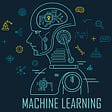## Baseline Model #2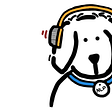## Semantic Segmentation using CNN’s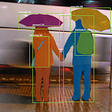## Design of Image Recognition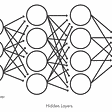## Machine Learning : Understanding Our Language Part I## [Lecture Notes] What is Softmax?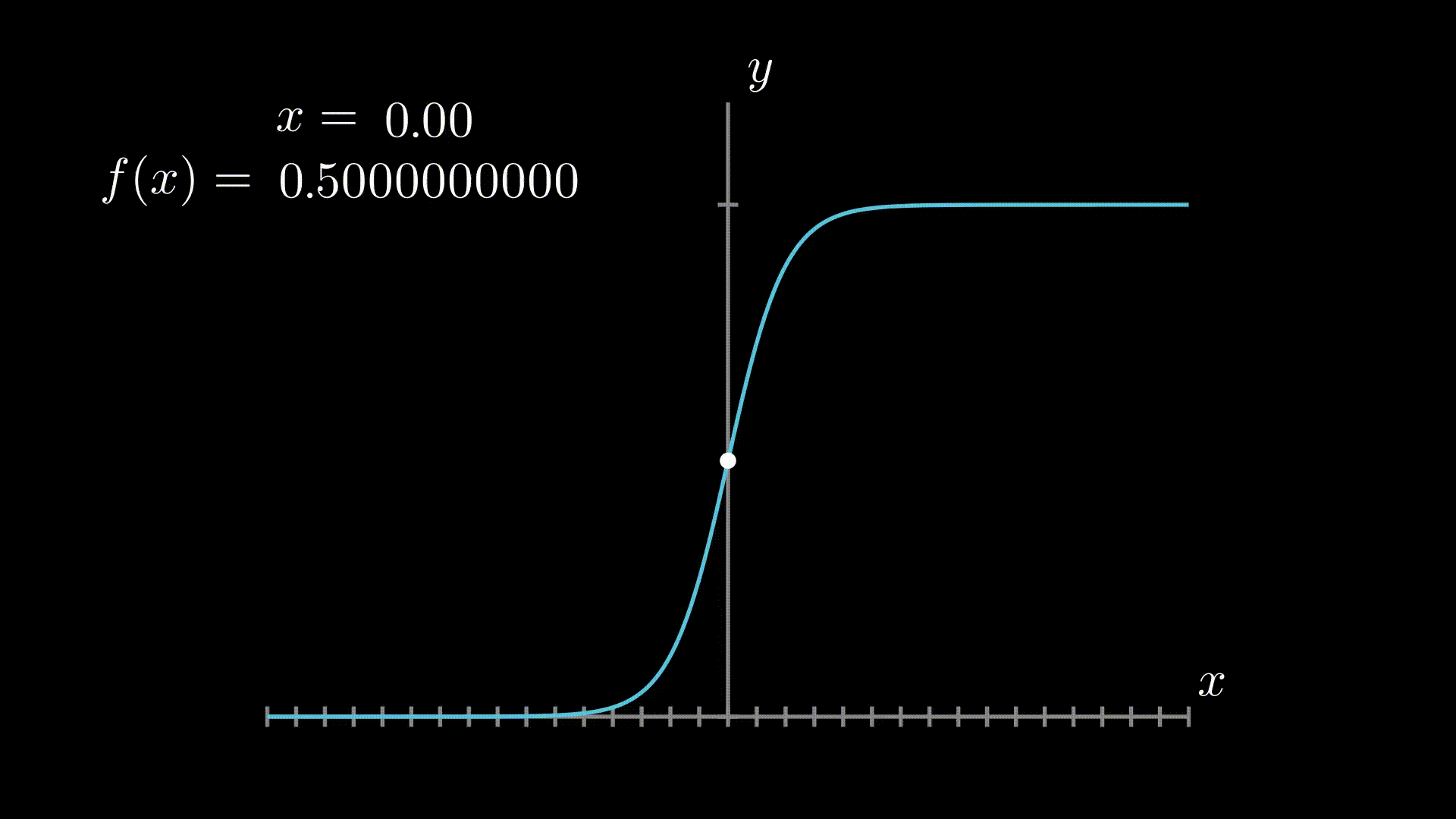## A New Way to BOW Analysis & Feature Engineering — Part2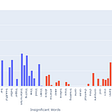## Implementing K Nearest Neighbors from scratch in Python## Li Yin

AI consulting and freelancing. Ex AI researcher@ Meta AI. Github:https://github.com/liyin2015.

## An Analytical Look Into Deep-Q Networks (DQNs)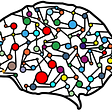## Searching for lottery tickets inside large neural networks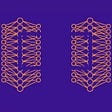## Variational Autoencoder : Intuition and Implementation## What is a Recurrent Neural Network?